# hs-minor-mode中对verilog-mode的支持？

``````begin
...
end
``````
``````case(a)
...
endcase
``````
``````module module0(...)
...
endmodule
``````

``````(defvar hs-special-modes-alist
(mapcar 'purecopy
'(
(verilog-mode "begin" "end" "/[*/]" nil nil)
)
)
)
``````

1. 写法不对

`hs-special-modes-alist` 是 HideShow 的变量，如果你先定义了，那么 HideShow 的定义无效；如果 HideShow 先加载，你的定义无效。

1. 用法不对

Verilog 其实已经向 `hs-special-modes-alist` 添加了规则，`begin...end` 是可以折叠的，但是光标位置要在 `begin``b` 字母 或 `end` 任意字母上，或者光标在 `begin...end` 之外

1. 添加对 `case/module` 的支持：
``````(setq hs-special-modes-alist
(cons '(verilog-mode "\\<\\(begin\\|case\\|module\\)\\>"
"\\<\\(end\\|endcase\\|endmodule\\))\\>"
nil verilog-forward-sexp-function)
hs-special-modes-alist))
``````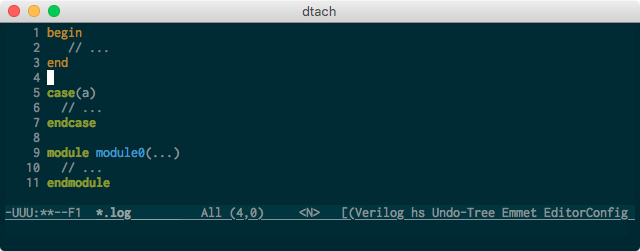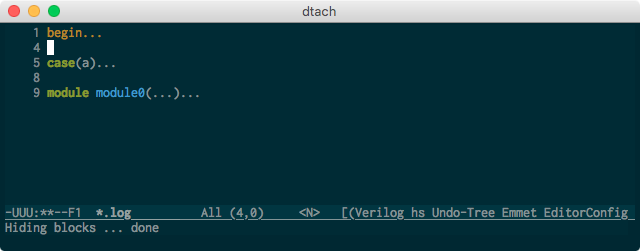``````(setq hs-special-modes-alist
(cons '(verilog-mode "\\<\\(begin\\|case\\|module\\)\\>"
"\\<\\(end\\|endcase\\|endmodule\\))\\>"
nil verilog-forward-sexp-function)
hs-special-modes-alist))
``````

``````module adder1(x,y,sum,cin,cout);
input x,y,cin;
output sum,cout;

assign sum = x^y^cin;
assign cout = (x&y)^(cin&(x^y));
``````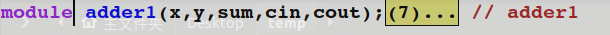但是，如果我这样写，他的Hide起来就有点诡异了：

``````module adder1(
input  x,
input  y,
input  cin,

output sum,
output cout
);
assign sum = x^y^cin;
assign cout = (x&y)^(cin&(x^y));
``````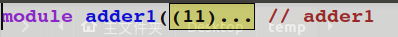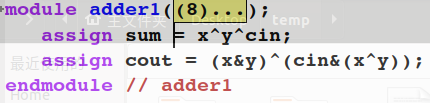``````begin
...
end
``````

hide之后留下了个

``````begin
...
``````

1. 并没有多出括号

``````module adder1(
input  x,
input  y,
input  cin,

output sum,
output cout
);
assign sum = x^y^cin;
assign cout = (x&y)^(cin&(x^y));
``````

折叠之后当然是：

``````module adder1(...// adder1
``````

因为你这个函数第一行就是 `module adder1(`

2. `...` 并不奇怪

`...` 表示折叠的内容，没有 `...` 你怎么知道有折叠。你也可以改成其他的符号。

3. 修改之前的 `()` 被折叠是误打误撞

你那边可能有其他折叠规则，刚好匹配到了`()`。虽然参数表折叠了，但是函数体没有折叠，这是错的。

``````if (a == b)
{
a++;
b++;
}
``````

``````if (a == b) {...}
``````

``````if (a == b}
{...
``````

``````"\\(\\<begin\\>\\|\\<case\\>\\|\\<module\\>\\)"
"\\(\\<end\\>\\|\\<endcase\\>\\|\\<endmodule\\>\\)"
``````

``````if (a == b)
{
a++;
b++;
}
``````

``````if (a == b)
{...}
``````